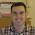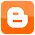## Thursday, December 27, 2012

### A JavaScript Quiz and a JavaScript Quiz Engine

Here is a very simple JavaScript quiz for you to try and have some fun with!
For this quiz, I wrote a very simple quiz engine using JavaScript and some JQuery APIs. To create a quiz all you need to do is create a JSON object which contains Question / Answer info and pass it to a single API. The engine will:
• determine if a question has a single answer or multiple answer and show radio buttons or check boxes accordingly.
• display user progress
• display results and correct / incorrect questions.
Source code is on my GitHub. I have also put the files on github pages. So if you want a quiz on your site, import the engine by simply importing the CSS and the JavaScript (ensure you also have JQuery being pulled in from somewhere).
```

```
Then create a JSON object of your questions and answers. The JSON object is an array of Question / Answer. Each element in the array consist is a map of three elements:
• The question
• The possible answers (the answers to present to the user) - specified as an ordered array.
• The correct answer
For example:
```var quiz_questions = {questions:[
{"question":"Which of the following is a risk of using Constructor function?",
"answers":["If it is invoked without using `new` keyword, the `this` variable is not bound to local object but to the global namespace.", "Performance risk", "There are no risks"],
...]
```
The correct answer is a numeric value, which represents the position in the list of the possible answers. If there is more than one correct answer, separate the correct answers by commas. For example:
```{"question":"Which of the following is true about functions?",
"answers":["Functions can be expressed as literals",
"Functions can be assigned to variables, array entries and properties of other objects",
"Passed as arguments to other functions",
"Returned as values from functions",
"Can have properties created and assigned dynamically",
"Can solve climate change?"],
```
The engine will use check boxes for questions that have multiple answers and radio boxes for questions that have a single answer. To invoke the engine, it is just one API.
```quizModule(quiz_questions, \$('#intro'));
```
In this case `\$('#intro')` is the dom element I want the quiz to sit below. `quiz_questions` is the JSON object containing the question / answer pairings.

There is no server request handling whatsoever after the quiz loads. Everything happens client side from the first question to the generation of results.

If you have any interesting JavaScript questions send them on and I'll add them to the quiz! Or use the engine and create your own quiz.

1.Hi Alex Staveley
i am not technical person,
so i can't understand this
but i need this script for my General Knowledge Blog
so,
can you explain more?

2.Multiple answers? How would I do this?

3.If you specify more than one correct answer in the JSON the engine detects the question has multiple answers and does everything for you.

4.Hi is it possible for me to use sounds or images in the question as well as answers?

5.tanx dude tanx alot.......